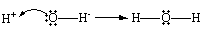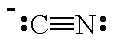# How can I classify each species as either a Lewis acid or a Lewis base for BeCl_2, OH^-, B(OH)_3 and CN^-?

Dec 3, 2014

You draw their Lewis structures and then make a decision based on possible reactions.

A substance may be either a Lewis acid or a Lewis base, depending on the reaction. But you can often make a good prediction.

BeCl₂

The Lewis structure for BeCl₂ isWe see that the Be atom has only four electrons in its valence shell. Be has an incomplete octet.

The Be atom will accept electrons to complete its octet.So BeCl₂ is a Lewis acid.

OH⁻

The Lewis structure of OH⁻ isThe O atom has three lone pairs of electrons. It can donate these electrons to form a bond to another atom.OH⁻ is a Lewis base.

B(OH)₃

The Lewis structure of B(OH)₃ isThe B atom has an incomplete octet, so it can act as a Lewis acid.

HO⁻ + B(OH)₃ → [HO-B(OH)₃]⁻

B(OH)₃ is also an oxyacid. So the H atom can also act as a Lewis acid.

HO⁻ + H-O-B(OH)₂ → H₂O + ⁻O-B(OH)₂

However you look at it, B(OH)₃ is a Lewis acid.

CN⁻

The Lewis structure of CN⁻ isThe C atom has both a lone pair and a negative charge, so it will behave as a Lewis base.

N≡C:⁻ + CH₃-Br → N≡C-CH₃ + Br⁻

Here’s a video on how to identify Lewis acids and bases.